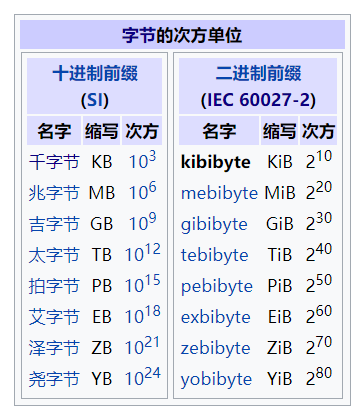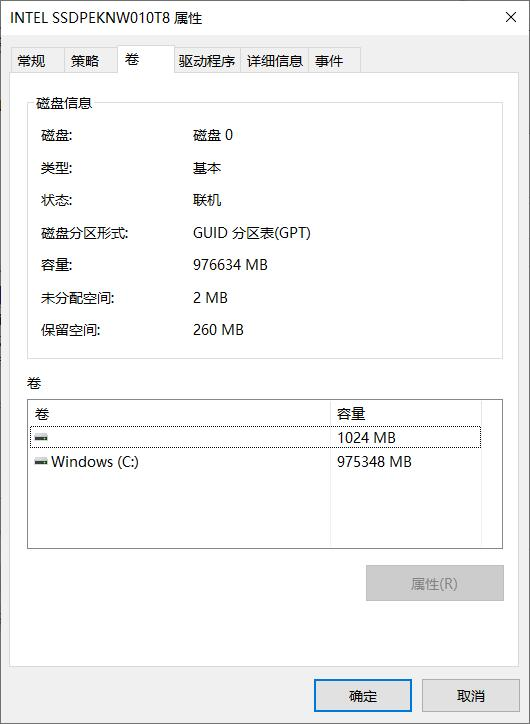## “数据” 与 “信息”

“通信”过程时时刻刻都在发生，你的肉眼看到的每一个画面，耳朵听到的每一点声音，神经传来的每一丝冲动，或多或少都在传递着信息，为你的大脑减少对外界的不确定性，辅助你的每一个决策。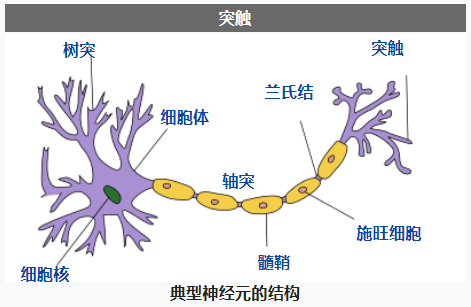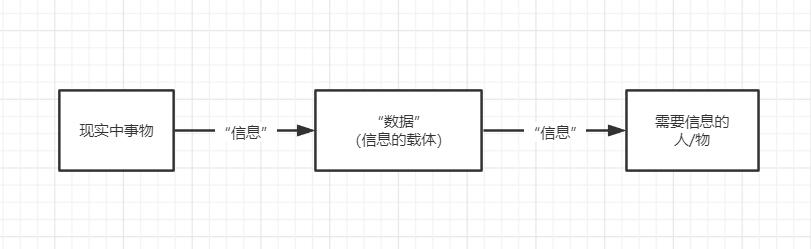## 香农与信息的度量

1948年，美国数学家 克劳德·香农 发表了论文《通信的数学原理》，奠定了信息论的基础，确定了更上一层讨论发展赖以生存的根基。目前计算机领域有关信息处理的一切，都是在香农信息论的框架之下进一步发展出来的。$$H(X)=\sum_{i}P(x_i)I(x_i)=-\sum_{i}P(x_i)log_bP(x_i)$$

$X$ 为一个随机事件，可能有 $x_1$, $x_2$, ..., $x_n$ 这 $n$ 种情况
$P(x_i)$ 为 $x_i$ 发生的概率，$I(x_i)$ 为 $x_i$ 发生对应的信息量
$b$ 为对数的底

## 抛硬币问题

1. 可能是正面
2. 可能是反面

$X$：抛一枚硬币，正反面两种情况 $x_0$, $x_1$
$P(x_0) = P(x_1) = \frac{1}{2}$
$b = 2$：我们用 bit 作为单位

$$H(X) = \sum_{i=0}^{1} P(x_i) (-log_2 P(x_i)) \\ = \sum_{i=0}^{1} \frac{1}{2} *(-log_2 \frac{1}{2}) \\ = \frac{1}{2} + \frac{1}{2} \\ = 1 \space bit$$

## 计算机的抛硬币模型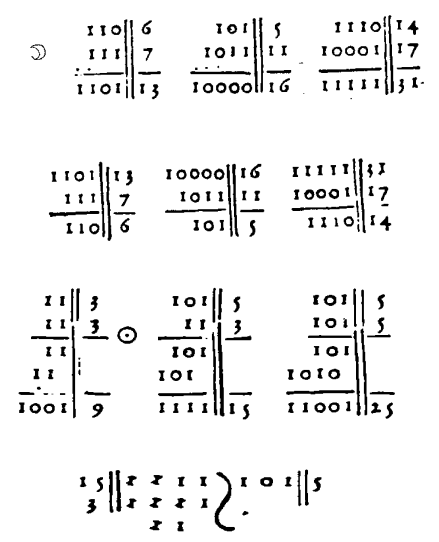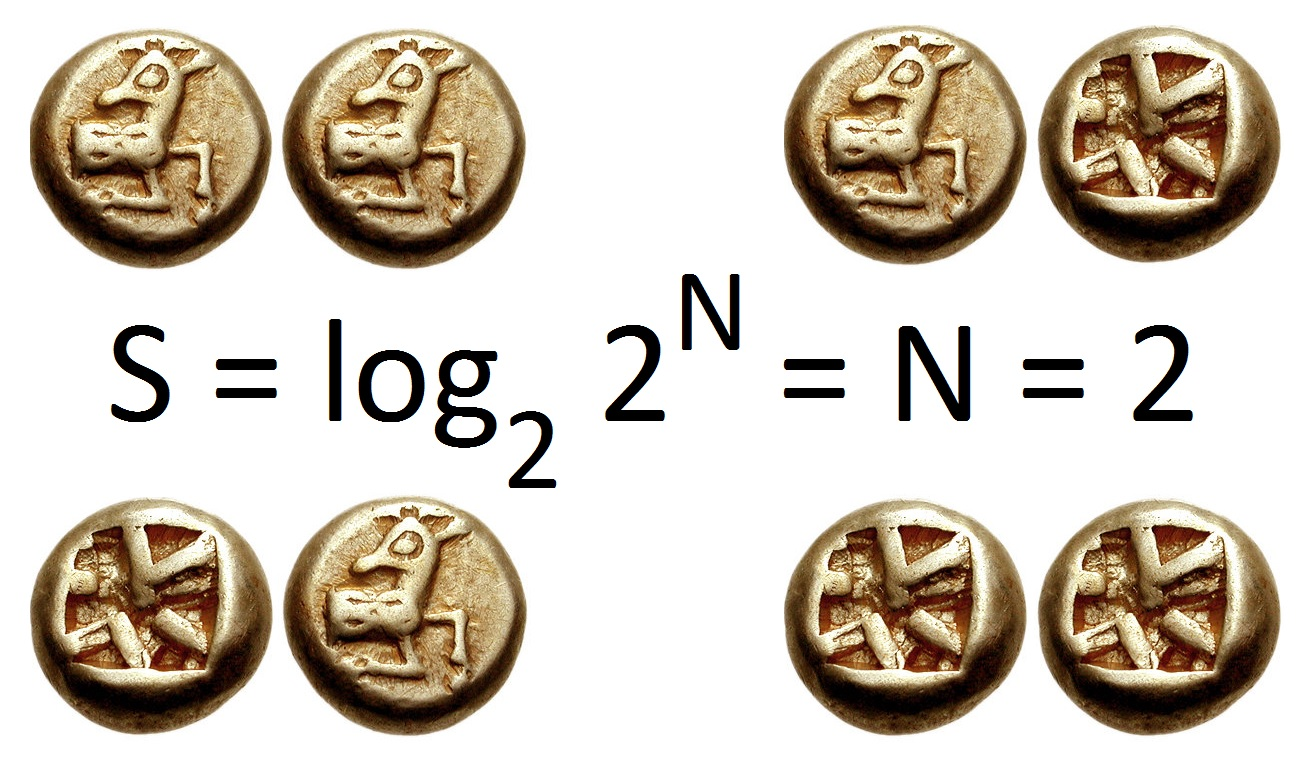0
1
2
3
4
5
6
7

## 英文字符的表示ASCII 全称 American Standard Code for Information Interchange (美国信息交换标准代码)，它设计了现代英语字符与电脑的”硬币体系“的对应关系，它从1963年第一版发布到1986年最后一次更新，一共定义了 128 个字符。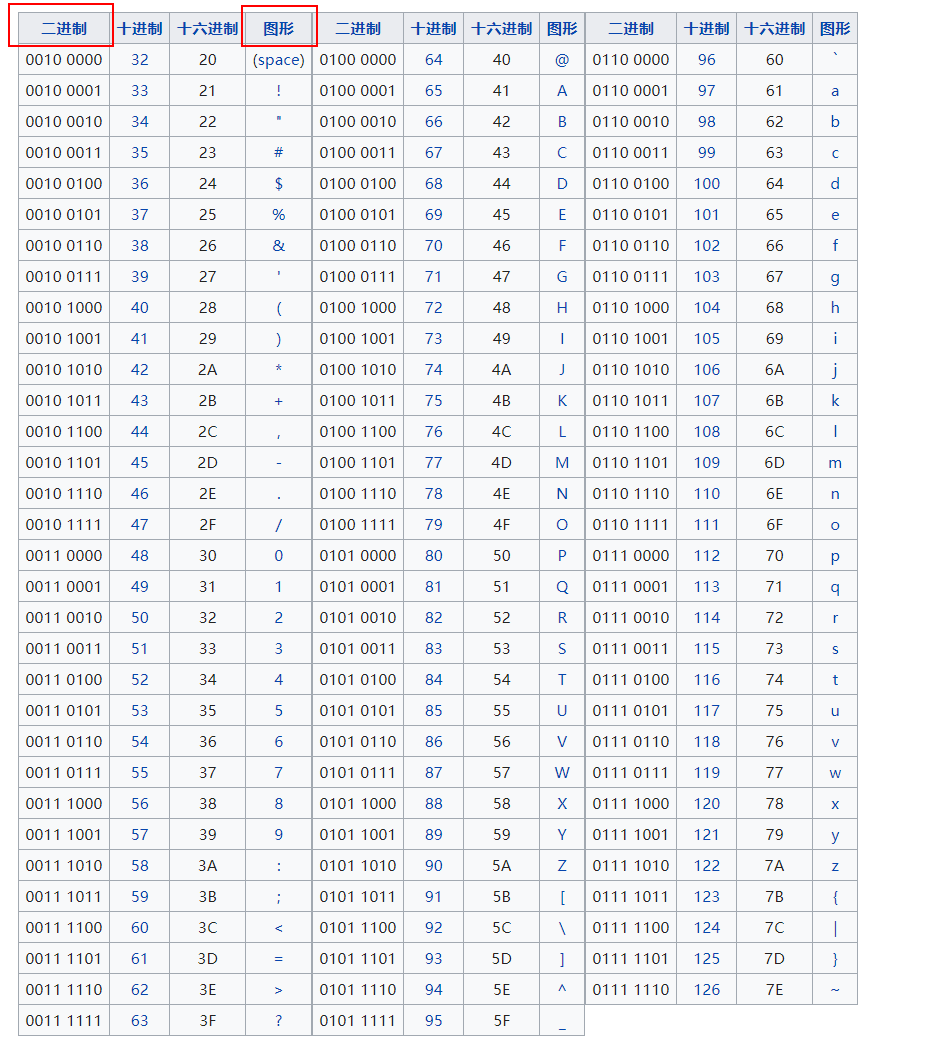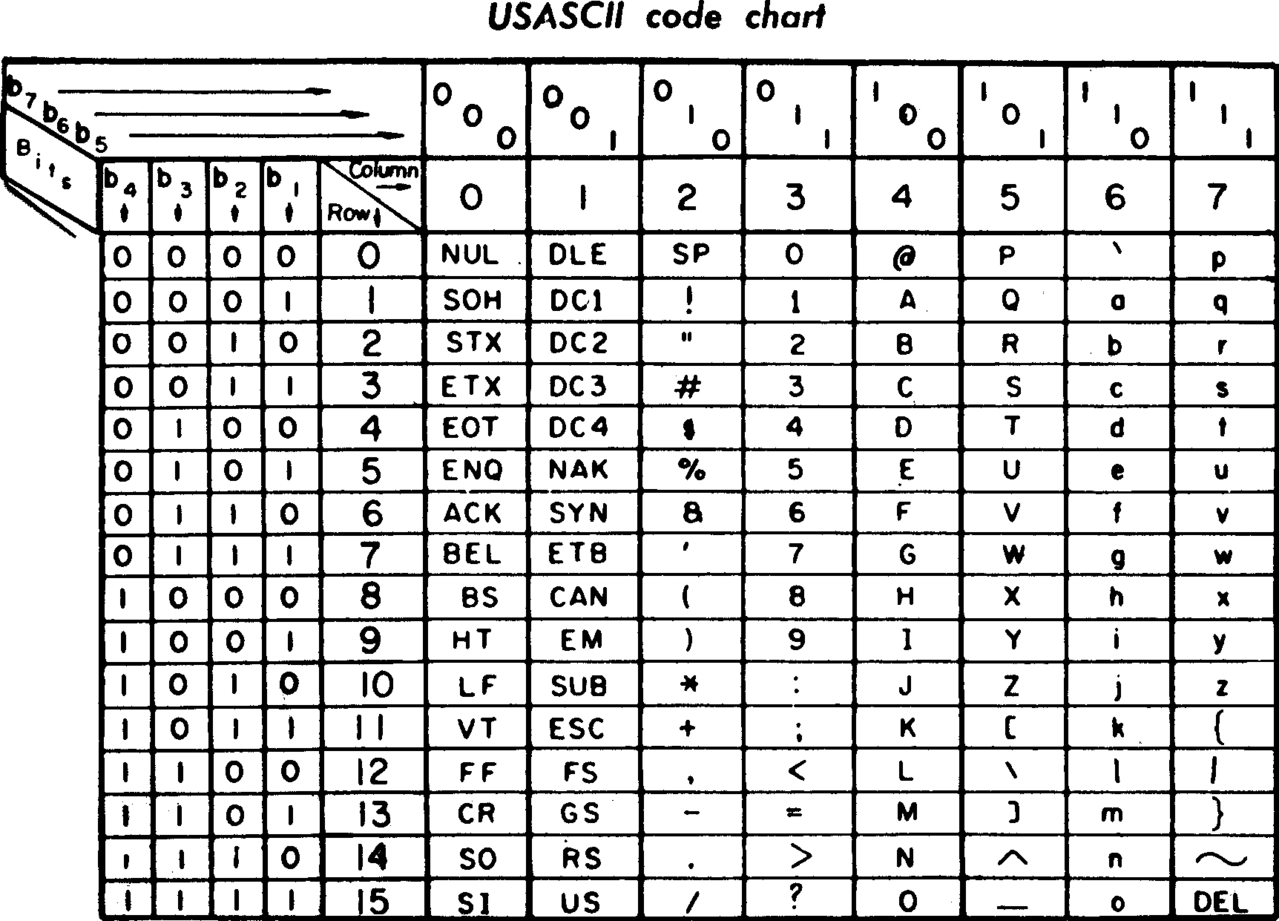1. 计算机在硬件上实现稳定的“二进制-硬币体系”
2. 使用者为体系中硬币组成的各个状态赋予意义
3. 根据意义，将现实数据转换为硬币角度的表示

## 数据量单位

KBKiloByte，千字节，顾名思义，1KB = 1000B
MBMegaByte，兆字节，1MB = 1000 KB = 1000,000B
GBGigaByte，吉字节，1GB = 1000 MB = 1000,000KB = 1000,000,000B
TBTeraByte，太字节，以此类推...

### 二进制与十进制单位

1000 这个倍数并不容易简单地通过移位操作（增加或减少“硬币”）的方式来实现，在机器角度实现复杂度要高许多；而 $2^{10} = 1024$，约等于 1000，恰好是移动 10 个二进制位的事情，所以人们也常用 1024 来做换算。这种方法暂时没找到合适的定义，这里我把它称为“千位二进制进位法”。